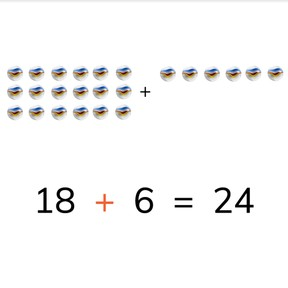# Addition to 30 making ten8,000 schools use Gynzy92,000 teachers use Gynzy1,600,000 students use Gynzy

## General

Students learn to add to 30 making tens.

2.OA.A.1
2.NBT.B.5

## Relevance

It is important to know how to add to 30 so you can determine totals of larger amounts.

## Introduction

Start by asking students where a number belongs on the number line. And then ask students to calculate the total of an addition problem to 20 on the number line.

## Development

First discuss the importance of addition to 30 with the students and explain how to add to 30 making tens. The learning goal is then presented in three ways, visually, in the abstract, and as story problems. You can select which you want to use via the menu in the bottom right. Alternatively you can begin with the visual explanation. Explain, using the images how you can add to 30 by counting on. Next discuss the abstract addition problems. Tell students that they can solve these by making ten and then adding on. They can also imagine a number line to support their addition. Sometimes you can already solve the problem without making tens or imagining the number line. Finally, discuss the steps of solving a story problem.

To check that students understand addition to 30 making tens, you can ask the following questions:
- Why is it useful to be able to add to 30?
- How do you make tens in an addition problem?

## Guided practice

Students first practice addition problems with visual support, then without visual support, and finally they are given a story problem.

## Closing

Ask students to do the two money problems that are on the interactive whiteboard.

## Teaching tips

Students who have difficulty adding to 30 or making tens can be supported by using the number line or by the use of manipulatives like blocks.

## Instruction materials

Optional: blocks

### The online teaching platform for interactive whiteboards and displays in schools

• Save time building lessons

• Manage the classroom more efficiently

• Increase student engagement